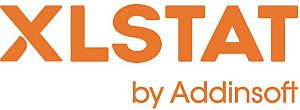# XLSTAT - Two sample comparison of variances

## Principle of two-sample comparison of variances tests

Take a sample S1 comprising n1 observations with variance s1². Take a second sample S2 comprising n2 observations with variance s2². XLSTAT offers three tests for comparing the variances of the two samples.

## Two-sample comparison of variances tests in XLSTAT

Three parametric tests are offered for the comparison of the variances of two independent samples.

### Fisher's F-test

Let R be the assumed ratio of the variances (R is 1 when equality is assumed).

The test statistic F is given by:

F = s1² ⁄ R*s2²

This statistic follows a Fisher distribution with (n1-1) and (n2-1) degrees of freedom if both samples follow a normal distribution.

Three types of test are possible depending on the alternative hypothesis chosen:

• two-tailed test,
• left-tailed test,
• right-tailed test.

### Levene's test

Levene's test can be used to compare two or more variances. It is a two-tailed test with the following hypotheses:

• H0 : s1² = s2²
• Ha : s1² ≠ s2²

The statistic from this test is more complex than that from the Fisher test and involves absolute deviations at the mean or at the median. The use of the mean is recommended for symmetrical distributions with averagely thick tails. The use of the median is recommended for asymmetric distributions.

The Levene statistic follows a Fisher’s F distribution with 1 and n1+n2-2 degrees of freedom.

### Bartlett’s homogeneity of variances test

Bartlett's test can be used to compare two or more variances. This test is sensitive to the normality of the data. In other words, if the hypothesis of normality of the data seems fragile, it is better to use Levene's or Fisher's test. On the other hand, Bartlett's test is more powerful if the samples follow a normal distribution.

This is also a two-tailed test similar to the Levene's test.
Bartlett's statistic follows a Chi-square distribution with one degree of freedom.This analysis is available in the XLStat-Basic addin for Microsoft ExcelKovach Computing Services (KCS) was founded in 1993 by Dr. Warren Kovach. The company specializes in the development and marketing of inexpensive and easy-to-use statistical software for scientists, as well as in data analysis consulting.

### Get in Touch

• Email:
sales@kovcomp.com